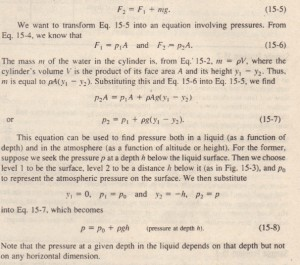# fluids at Rest

fluids at Rest

Figure I5-2a shows a tank of water-or other liquid-open to the atmosphere. As every diver knows, the pressure increases with depth below the air- water interface.The diver’s depth gauge. in fact.  a pressure sensor much like that of Fig. 15-1 h.  As every mountaineer knows. the pressure decreases with altitude as one ascends into the atmosphere. The pressures encountered by the driver and the mountaineer are usually called hydrostatic pressures. because- they are due to fluids that are static (at rest). Here we want to find an expression for hydrostatic pressure as a function of depth or altitude.  Let us look first at the increase in pressure with depth below the water’s surface.We set up a vertical)’ axis in the tank. with its origin at the air-water interface and the positive direction upward. We next consider a water sample contained in an imaginary right circular cylinder of horizontal base (or face) area A, such that Yl and

Yl (both of which are negative numbers) are the depths below the surface of the upper and lower cylinder faces, respectively.  shows a free-body diagram for the water in the cylinder. The waters in static equilibrium: that is, it is stationary and the forces on it balance. Three forces act on it vertically: Force FI acts at the toe. surface of the cylinder and is due to the water above the cylinder. Similarly, force F2 acts at the bottom surface of the cylinder and is due to the water below the cylinder. The gravitational force on the water in the cylinder is represented by mg, where m is the mass of the water in the cylinder. The balance of these forces is written as.The pressure at a point in a fluid in static equilibrium depends on the depth of that point but not on any horizontal dimension of the fluid or its container.

Thus, Eq. 15-8 holds no matter what the shape of the container. If the bottom surface of the container is at depth h, then Eq. 15-8 gives the pressure P there. In Eq. 15-8. P is said to be the total pressure or absolute pressure at level 2. I Too see why. note in Fig. 15-3 that the pressure P at level 2 consists of two contributions: (I) Po. the pressure due to the atmosphere. which bears down on the liquid,  nd (2) PG, the pressure due to the liquid above level 2. which bears down on level  2. In general. the difference between an absolute pressure and an atmospheric pressure called the gauge pressure, (The name comes from the use of a gauge to measure this difference in pressures.) For the situation of Fig. 15-3, the gauge pressure is pgh. Equation 15-7 also holds above the liquid surface: It gives the atmospheric pressure at a given distance above level I in terms of the atmospheric pressure PI at level I (assuming that the atmospheric density is uniform over that distance). For example, to find the atmospheric pressure at a distance d above level 1 inSample ProblemSample Problem